# Maximum/minimum problem

Karol

## Homework Statement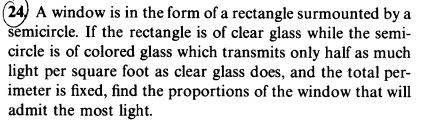## Homework Equations

Maxima/minima are where the first derivative is 0

## The Attempt at a Solution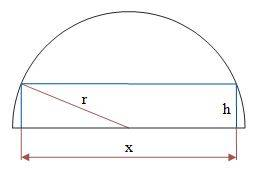$$r^2=\frac{x^2}{4}+h^2~\rightarrow~h^2=r^2=\frac{x^2}{4}$$
S will be the strength of the light through the whole opening: the semicircle and the rectangle inside.
I take the strength of light through of light through the rectangle as 1 and through the colored glass as ##~\frac{1}{2}##.
$$S=\frac{1}{2}(\text{area of a semicircle})+\frac{1}{2}hx$$
$$S=\frac{1}{2}\left[ \frac{\pi r^2}{2}+hx \right],~~S'=\frac{1}{2}\left[ \left( -\frac{2x}{4} \right)x+h \right]$$
$$S=\frac{1}{2}\left[ \left( r^2-\frac{x^2}{4} \right)-\frac{x^2}{2} \right],~~S'=0:~~\rightarrow~~x^2=-4r^2$$

If i try the ##~GM \leq AM~##: ##~\sqrt{h^2x^2}\leq \frac{h^2+x^2}{2}##
In order to maximize the left side equality is when the elements are equal: ##~x^2=h^2~\rightarrow~x^2=h^2-\frac{x^2}{4}~\rightarrow~x^2=\frac{4r^2}{5}##

Homework Helper
Dearly Missed

## Homework Statement

View attachment 221827

## Homework Equations

Maxima/minima are where the first derivative is 0

## The Attempt at a Solution

View attachment 221828
$$r^2=\frac{x^2}{4}+h^2~\rightarrow~h^2=r^2=\frac{x^2}{4}$$
S will be the strength of the light through the whole opening: the semicircle and the rectangle inside.
I take the strength of light through of light through the rectangle as 1 and through the colored glass as ##~\frac{1}{2}##.
$$S=\frac{1}{2}(\text{area of a semicircle})+\frac{1}{2}hx$$
$$S=\frac{1}{2}\left[ \frac{\pi r^2}{2}+hx \right],~~S'=\frac{1}{2}\left[ \left( -\frac{2x}{4} \right)x+h \right]$$
$$S=\frac{1}{2}\left[ \left( r^2-\frac{x^2}{4} \right)-\frac{x^2}{2} \right],~~S'=0:~~\rightarrow~~x^2=-4r^2$$

If i try the ##~GM \leq AM~##: ##~\sqrt{h^2x^2}\leq \frac{h^2+x^2}{2}##
In order to maximize the left side equality is when the elements are equal: ##~x^2=h^2~\rightarrow~x^2=h^2-\frac{x^2}{4}~\rightarrow~x^2=\frac{4r^2}{5}##

As I read the problem you have a rectangle of height ##h## and width ##2r##, surmounted by a semi-circle of radius ##r##. The total glass area is ##A = A_2 + A_s##, where ##A_r## is the rectangular area and ##A_s## is the semicircular area. What formulas (in terms of ##r## and ##h##) would you use to get ##A_r, A_s?##
The perimeter is ##P = 2h + 2r + \pi r.## The light admitted is proportional to ##F =A_r + (1/2) A_s##, and you want to maximize ##F## subject to constant ##P##.

Other interpretations: perhaps the author intends "perimeter" to mean total perimeter of both glass pieces, in which case it would be ##2h + 6r + \pi r,## or perhaps the glass individual glass pieces are to be surrounded by a frame, and "perimeter" means the total length of all material in the frame. In that case it would be##2h + 4r + \pi r.## However, my guess is that he/she means the original interpretation ##2h + 2r + \pi r.## That is just the total length of the outside edges.

Last edited:
Gold Member
S=12(area of a semicircle)+12hxS=12(area of a semicircle)+12hx​
That equation states that all light goes through a semicircular area, and intensity is cut in half.
And that more light goes through a clear rectangular area, and intensity is also cut in half, contrary to the problem statement.
As I understand it, light enters a semicircular area, part of which is taken up by a clear window, and the rest taken up by colored glass.

I take that the perimeter of the clear glass is fixed, and is equal to 2x + 2h as one of the boundary conditions.

Karol
This:
$$S=\frac{1}{2}(\text{area of a semicircle})+\frac{1}{2}hx$$
Isn't a mistake. ifi interpret correctly, according to my drawing, then the light from the rectangular window adds to the light from the semicircle.
I took the whole semicircle.
Ray, your ##~2h + 4r + \pi r.~## means: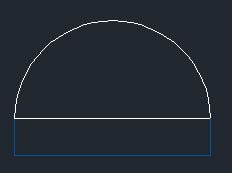The rectangle isn't surrounded and it should be

#### Attachments

Homework Helper
Dearly Missed
This:
$$S=\frac{1}{2}(\text{area of a semicircle})+\frac{1}{2}hx$$
Isn't a mistake. ifi interpret correctly, according to my drawing, then the light from the rectangular window adds to the light from the semicircle.
I took the whole semicircle.
Ray, your ##~2h + 4r + \pi r.~## means:
View attachment 221891 The rectangle isn't surrounded and it should be

No, that is not what it means. The perimeter of the rectangle is ##2h + 2(2r) = 2h + 4r## because each side has length ##h## and the top and bottom each have length ##2r## (which is the diameter of the semicircle).

Homework Helper
Gold Member
View attachment 221891 The rectangle isn't surrounded and it should be
@Karol: "Surrounded" and "surmounted" don't mean the same thing. This is a typical problem seen in many calculus texts, and in my opinion "surmounted" means the semicircular window is on top of the rectangle shaped one, and the "perimeter" means the outside perimeter. I could be wrong. (But I don't think so).

•SammyS
Karol
So it's: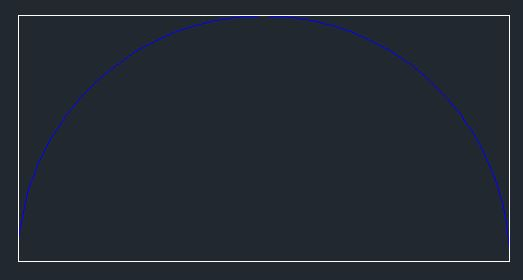Then the total glass area isn't ##~A=A_r+A_s##
Is a semicircle always exactly half a circle? if so then the proportions are fixed, h is always equal to r

#### Attachments

Staff Emeritus
Homework Helper
Gold Member
So it's:
View attachment 221930 Then the total glass area isn't ##~A=A_r+A_s##
Is a semicircle always exactly half a circle? if so then the proportions are fixed, h is always equal to r
I could barely see the detail in this figure. I changed brightness values to get: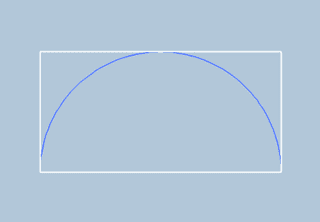This is not the correct sketch for your problem.

Your figure in post #4 is the correct one.The perimeter for this window in ##\ 2r+2h+\pi r \ ##.

#### Attachments

Karol
How did you change the brightness of the jpeg, with which program?
The intensity of light F is proportional to:
$$F=A_r+\frac{1}{2}A_s=2(hr)+\frac{1}{2}\left[ \frac{1}{2}\pi r^2 \right]$$
I will denote the fixed perimeter as ##~K=2h+2r+\pi r##
$$F=(K-2r-\pi r)r+\frac{1}{4}\pi r^2$$
$$F'=K-2r-\pi r+r(-2-\pi)+\frac{\pi}{2}r=K-\left[ 2(\pi+2)-\frac{\pi}{2} \right]K$$
$$F'=0:~r=\frac{2K}{3\pi+8}~\rightarrow~h=\frac{(\pi+4)K}{2(3\pi+8)}$$
The relation:
$$\frac{h}{r}=\frac{\pi+4}{4}$$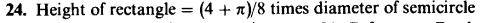#### Attachments

Staff Emeritus
Homework Helper
Gold Member
How did you change the brightness of the jpeg, with which program?
...
I inserted a copy of your figure into a blank MS Word (2010) document. (That graphic of yours is actually in PNG format.)

I then used Picture Tools in MS Word to alter the contrast as well as the brightness.

Finally, I used the Snipping Tool from Windows 7 to get the resulting PNG file which I uploaded to PF and Post #8 above.

Karol
Thank you Ray, 256bits, LCKurtz and SammyS